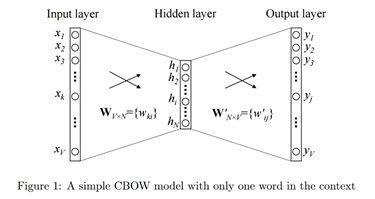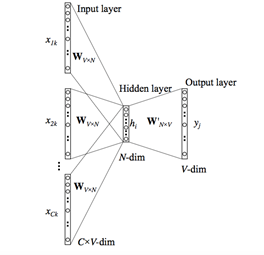# Global Data Science Forum

View Only

### Word Embedding

#### By Moloy De posted Thu August 13, 2020 09:25 PM

Word embedding is one of the most popular representation of document vocabulary. It is capable of capturing context of a word in a document, semantic and syntactic similarity, relation with other words, etc. Loosely speaking, they are vector representations of a particular word. Having said this, what follows is how do we generate them? More importantly, how do they capture the context?

Word2Vec is one of the most popular technique to learn word embeddings using shallow neural network. It was developed by Tomas Mikolov in 2013 at Google.

Consider the following similar sentences: Have a good day and Have a great day. They hardly have different meaning. If we construct an exhaustive vocabulary, let’s call it V, it would have V = {Have, a, good, great, day}. Now, let us create a one-hot encoded vector for each of these words in V. Length of our one-hot encoded vector would be equal to the size of V = 5. We would have a vector of zeros except for the element at the index representing the corresponding word in the vocabulary. That particular element would be one. The encodings below would explain this better.

Have = [1,0,0,0,0]; a=[0,1,0,0,0]; good=[0,0,1,0,0]; great=[0,0,0,1,0]; day=[0,0,0,0,1]. If we try to visualize these encodings, we can think of a 5-dimensional space, where each word occupies one of the dimensions and has nothing to do with the rest, like no projection along the other dimensions. This means ‘good’ and ‘great’ are as different as ‘day’ and ‘have’, which is not true.

Our objective is to have words with similar context occupy close spatial positions. Mathematically, the cosine of the angle between such vectors should be close to 1, i.e. angle close to 0.Here comes the idea of generating distributed representations. Intuitively, we introduce some dependence of one word on the other words. The words in context of this word would get a greater share of this dependence. In one hot encoding representations, all the words are independent of each other, as mentioned earlier.

Word2Vec is a method to construct such an embedding. It can be obtained using two methods both involving Neural Networks: Skip Gram and Common Bag Of Words (CBOW)

CBOW Model: This method takes the context of each word as the input and tries to predict the word corresponding to the context. Consider our example: "Have a great day". Let the input to the Neural Network be the word, "great". Notice that here we are trying to predict a target word "day" using a single context input word great. More specifically, we use the one hot encoding of the input word and measure the output error compared to one hot encoding of the target word "day". In the process of predicting the target word, we learn the vector representation of the target word.

Let us look deeper into the actual architecture.The input or the context word is a one hot encoded vector of size V. The hidden layer contains N neurons and the output is again a V length vector with the elements being the softmax values.

Here:

• Wvn is the weight matrix that maps the input x to the hidden layer (V*N dimensional matrix)
• W`nv is the weight matrix that maps the hidden layer outputs to the final output layer (N*V dimensional matrix)

The hidden layer neurons just copy the weighted sum of inputs to the next layer. There is no activation like sigmoid, tanh or ReLU. The only non-linearity is the softmax calculations in the output layer. But the above model used a single context word to predict the target. We can use multiple context words to do the same.The above model takes C context words. When Wvn is used to calculate hidden layer inputs, we take an average over all these C context word inputs.

So, we have seen how word representations are generated using the context words. But there’s one more way we can do the same. We can use the target word whose representation we want to generate, to predict the context and in the process, we produce the representations. Another variant, called Skip Gram model does this.

Skip-Gram model:This looks like multiple-context CBOW model just got flipped. To some extent that is true. We input the target word into the network. The model outputs C probability distributions. For each context position, we get C probability distributions of V probabilities, one for each word.

In both the cases, the network uses back-propagation to learn. Both have their own advantages and disadvantages. According to Mikolov, Skip Gram works well with small amount of data and is found to represent rare words well. On the other hand, CBOW is faster and has better representations for more frequent words. To make the algorithm computationally more efficient, tricks like Hierarchical Softmax and Skip-Gram Negative Sampling are used.

REFERENCE: Introduction to Word Embedding and Word2Vec

QUESTION I: In general, how many dimensions do we would need to embed words in a corpus?

QUESTION II: How one uses back-propagation to train the models?

#Highlights-home
#Highlights